International Journal of Geomagnetism and Aeronomy
Vol. 4, No. 2, August 2003

# Method of estimation of the temperature seasonal variations in the lower thermosphere from the ionospheric data

G. V. Givishvili and L. N. Leshchenko

Institute of Terrestrial Magnetism, Ionosphere, and Radio Wave Propagation, Troitsk, Russia

### Abstract

A comparison of the latest versions of the empirical models of the atmosphere (MSIS) and ionosphere (IRI) in the 100-115 km altitude range for the conditions of moderate solar activity and middle latitudes is performed. Relations are derived allowing us to estimate the temperature seasonal variations in the lower thermosphere on the basis of the data on the electron concentration ne and/or the critical frequencies of the ionospheric E layer foE. It is demonstrated that the character of the annual variation of the temperature T and molecular oxygen concentration [O2] in the MSIS model contradicts the conclusions of the IRI model on a constancy during the year of the E -layer maximum height hmE and on the semiannual variations of the [NO+]/[O+2] ratio for the above-mentioned conditions.

### 1. Introduction

Givishvili and Leshchenko [ were the first to try to reveal seasonal features of the temperature long-term trends at the height of the E -layer maximum hmE on the basis of the electron concentration and/or the critical frequency foE measurements. However, the annual variations of the molecular oxygen concentration [O2], the principal ionized constituent at E -layer heights, have been taken into account by Givishvili and Leshchenko [ only indirectly, using the concentration ratio of the atomic oxygen O and molecular nitrogen N 2. The aim of this study is to consider more accurately factors determining the seasonal behavior of the electron production and loss rates in order to be able to analyze the fine structure of the seasonal variations of the long-term trends in the lower thermosphere temperature derived from ne or foE measurements.

### 2. Initial Equations

The critical frequency foE is related to ne by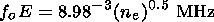(1)

The equilibrium electron concentration ne in the E region only slightly depends on diffusion processes, thermospheric winds, and electric fields. Therefore the formulae is correct there:(2)

where qm is the ionization rate in the layer maximum and aD is the effective recombination coefficient. The values of qm and aD both depend on T. This fact makes it possible to determine reliably values of T at the height of the E -layer maximum hmE using the measurements of foE if the qm and aD dependencies on T are known.

The ionization of the E region is provided mainly by the solar radiation in the l = 97.7 nm and (Lyman- b ) 102.6 nm lines interacting with oxygen molecules. This two channels provide formation of 75-85% of all charged particles. An additional source of ion formation at heights of 100-120 km is related to the solar X rays interacting with oxygen molecules and also nitrogen molecules and oxygen atoms. Thus about 80-90% of the ionization rate q depend on the O2 content in the lower thermosphere. Therefore q is determined by the Chapman equation [Chapman, 1931]: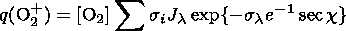(3)

where si and sl are the cross sections of ionization and absorption for an O2 molecule, respectively, c is the solar zenith angle, Jl is the radiation flux at the wavelengths 102.6, 97.7, and 1-8 nm. The photoionization cross-sections are 10-18, 2.510-18, and (0.2-3.6)10-18 for 102.6 nm, 97.7 nm, and 1-8 nm, respectively. The absorption cross-sections are 1.510-18, 4.010-18, and (0.2-0.9)10-18 for the same wavelengths, respectively [Samson and Cairns, 1964; Watanabe and Hinteregger, 1962]. All sl and si are constant and do not influence seasonal and long-term changes of the ionization rate. Therefore, to simplify the process of q (O2 ) calculations, we accept below generalized values si = 1.8210-18 cm 2 and sl = 2.3410-18 cm 2 taking into account the weighted input of the above-indicated solar radiation ranges into the ionization rate.

In the layer maximum where the dq( O2+)/dh = 0 condition [Chapman, 1931] is fulfilled we have(4)

Equation (4) is not convenient for calculations of T because of two reasons. First, one has to have all the information on absolute values and seasonal variations of [O2]. However different authors [Alcayde et al., 1974; Mayr et al., 1976; Scialmon, 1974] give significantly different information. Second, to know the seasonal variations of the height where the dq(O2+)/dh = 0 condition is fulfilled, that is, to which height the derived temperature corresponds, one has to perform additional calculations to reconstruct the q(h) vertical profile using formula (3).

So we rewrite formula (3) in the form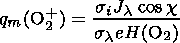(5)

Here H( O2) = kT/mg is the scale height for O2, k is the Boltzmann constant, m is the molecular weight of O2, and g is the gravity acceleration. Substituting numerical values of k, m, g, s1, and s2 into formula (5), we obtain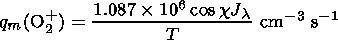(6)

Now there is no need to know the absolute values of [O2]. According to Ivanov-Kholodny and Firsov  the flux of the radiation ionizing O2 is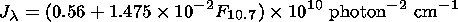where F10.7 is the solar activity index.

The loss of free electrons in the daytime quiet E region occurs in their dissociative recombination with NO+ and O2+ ions with the rate constants [Mehr and Biondi, 1969]: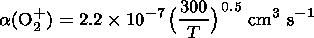(7)

and(8)

Since at the considered heights ne = [ NO+] + [ O2+] and a ( NO+)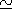2a ( O2+), the total loss rate is determined by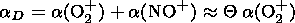(9)

where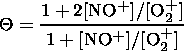Finally, we have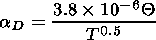(10)

Two ways to calculate the temperature from the foE data are considered. In the first, formula (4) is taken into account, the second is based on formula (6). Substituting these two formulae and formulae (1) and (10) into equation (2), we have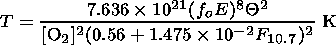(11)

and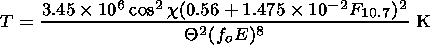(12)

### 3. Analysis of the Base Equations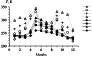Figure 1
According to the International Reference Ionosphere (IRI) model [Bilitza, 1997], the height of the E -layer maximum hmE is constant over the year and equal to 110 km. Therefore we took the concentrations of NO+ and O2+ ions (parameter Q) and the electron concentration ne (foE) for the altitude of 110 km needed to analyze formula (11). The calculations using formula (11) were performed for 9 vertical ionospheric sounding stations shown in Table 1, conditions of local noon (1200 LT) and moderate solar activity (F10.7 = 120). The period from September 1971 to August 1972 corresponds to this solar activity level. The data on the molecular oxygen concentration were taken from the MSIS (Mass-Spectrometer and Incoherent Scatter) model [Hedin, 1991]. Figure 1 shows the temperature values T(M) from this model for the coordinates of 4 out of 9 stations indicated above.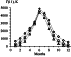Figure 2
Calculating the temperature T(11) by formula (11) for hmE lead to an absurd conclusion that the summer values of T(11) exceed the winter values by a factor of 10 or even a hundred (see Figure 2). At the same time they indicate that the hmE height can not stay fixed during the year: in summer the E -layer maximum should decent to the region of higher values of [O2].

To specify the character of the seasonal variations of qm and hmE and also to estimate the influence of the seasonal variations of the Q parameter on the temperature evaluation, we use formula (12). Following IRI we presume that hmE = 110 km all over the year. The results of the calculations for the four stations indicated above are shown in Figure 1 as T(12) values. One can see that in this case the deviations between T(12) and T( M) are relatively small in summer but are considerable in equinox periods. The important thing is that a semiannual component which is absent in the annual variations of T( M) is visually seen in the seasonal behavior of T(12).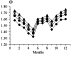Figure 3
This semiannual component of the T(12) seasonal behavior is due to the seasonal variations of the Q parameter (see (Figure 3)). In the IRI model these variations repeat the annual behavior of the NO+ concentration. Two features should be mentioned in this behavior: considerable semiannual variations of [NO+] and some excess of the winter values of [NO+] over the summer values. However, the data of the observations on board the Orbiting Geophysical Observatory (OGO 6) show that at 105 km the concentration of nitric oxide (the main photochemical source of the NO+ formation at middle latitudes) changes smoothly from the minimum values of about 107 cm-3 in winter to the maximum values of about 2.5107 cm-3 in summer [Gravens and Stewart, 1978]. At the same time, the seasonal behavior of the molecular oxygen concentration controlling the concentration of O+2 ions at heights of the E region is also rather monotonous. This concentration decreases from winter months to summer. Thus, there is no semiannual component in the annual behavior of the [NO+]/[ O+2] ratio and this ratio should be higher in summer than in winter. Therefore it is not clear what causes the seasonal variations of Q presented in the IRI model. There is only one source of data on the E -layer ion composition: the data of rocket mass-spectrometer measurements. Evidently, there are not enough of these data to derive statistically significant conclusions on the seasonal variability of the [ NO+]/[ O+2] ratio.Figure 4
Since the problem of existence and character of the Q seasonal variations needs a specification, we assumed that these variations are absent. We accepted this hypothesis as a first approximation and calculated new values of T(12)* under condition that Q =Qc, where Qc is an annual mean value of Q at each station considered. The results of the calculations shown in Figure 4 also do not correspond to the structure of the temperature annual variations in the E -layer maximum corresponding to the MSIS model: the obtained values of T(12)* are maximum in fall-spring months but not in summer. This result demonstrates that formula (12) should contain a function taking into account the seasonal variations of [O2].

Givishvili and Leshchenko [ introduced into formula (12) a parameter g which took into account the [O2] seasonal variations according to the data of the rocket measurements of the [O]/[N2] ratio at a height of 130 km [Antonova and Katyushina, 1976]. In other words, T was calculated using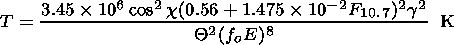(13)

The evaluation of g from the data on the [O]/[N2] ratio made it possible to estimate the annual T variations at hmE rather approximately. More explicit description of the relative seasonal variations of [O2] at height of the E -layer maximum provides the MSIS model, if one determines the g parameter in another way: as a ratio g = [ O2]i/[ O2]J, where J stands for January and i = 1-12 corresponds to the month number. Thus g is characterized by the relative changes of [O2] at about 110 km relative the values in January.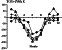Figure 5
Formula (13) was also analyzed for the conditions of the local noon (1200 LT) and moderate solar activity ( F10.7 = 120 ) for the coordinates of the same stations. The c values corresponded to the 15th day of each month. The data on foE were taken from the IRI model. Since according to this model the hmE height at noon is constant during the year and equal to 110 km, the annual variations of g should correspond to the annual behavior of [O2] at this height. The results of calculations are presented in Figure 5 as values DT = T(13)- T( M).

One can easily see that the discrepancy between T(13) and the model values of the temperature T( M) is in this case maximum in summer months when the discrepancy reaches 100-200 K. In winter months the difference between T(13) and T( M) is small. This indicates to the fact that in winter hmE is actually close to 110 km. In summer months it should decent down to the altitudes where [O2] is considerably higher than at 110 km, as has been suggested earlier. This conclusion agrees with empirical models of the midlatitude ionosphere [Fatkullin et al., 1981; Givishvili and Fligel', 1971; Robinson, 1960]. According to these models the noon values of hmE vary during the year from the maximum winter values equal to 108-112 km to the minimum summer values of about 104-108 km. The incoherent scatter measurements conducted in Kharkov (50o N) in 1978-1983 also indicate to a lowering of hmE from winter to summer [Ivanov-Kholodny et al., 1998]. To check the conclusion on variability of the hmE and to estimate its changes during the year, we calculated g ( M)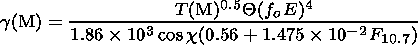(14)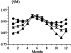Figure 6Figure 7
The results of the calculations are shown in Figure 6. They make it possible to see what concentrations of [O2] at 110 km correspond to the temperature T( M) from the MSIS model, foE from the IRI model, and the assumption that Q =  const. Further, using the ratio [O2]i/[ O2]J from the MSIS model, the heights of the E -layer maximum are found and denoted as hm( M) (see Figure 7). One can see that the hm( M) heights vary over the year in the way close to that found in the models [Fatkullin et al., 1981; Givishvili and Fligel', 1971; Robinson, 1960]. (It should be noted that according to Ivanov-Kholodny et al.  the amplitude of the annual variation of hmE at a latitude of 50o under F10.7 = 120 reaches about 7 km. According to our evaluations for the same latitude and moderate solar activity level the seasonal variations of hmE do not exceed ~3 km. This discrepancy is rather large, but it does not influence the general conclusion on the variability of hm( M) over the year.)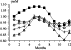Figure 8
Then, using the same ratios [O2]i/[ O2]J from MSIS the values of Tm( M) characterizing the temperature at the hm( M) height varying during the year are found. Substituting into equation (14) the values Tm( M) instead of T( M), we obtain the values of gm( M) corresponding to the variable hm( M) height (see Figure 8). Figure 8 shows also the values of g used by Givishvili and Leshchenko [. One can see that the latter are overestimated as compared with the values determined in this paper but by not more than by 10-15% depending on season and latitude. This fact does not influence the results of the above-mentioned paper which deals with the seasonal features of the long-term temperature trends, because the annual behavior of g in that paper on the whole does not contradict the MSIS model. Nevertheless, to use the vertical sounding data not only to derive the long-term temperature trends but also to evaluate its absolute values it is desirable to use formula (14).

Thus, we have now all the necessary information for calculation of the temperature at the heights of the E -layer maximum on the basis of the data on foE, including the data on the changes of both this very height (the hm( M) parameter) and the O2 concentration at this height, i.e., the gm( M) parameter.

As for the IRI model, it is worth mentioning that the reliability of the data on the electron concentration (frequency foE ) in it arises no doubts. Evidently, the same is not true for the seasonal variations of both the maximum height and ion composition of the E layer. The calculations performed show that the hmE height should vary over the year. However, the assumption on the absence of annual variations of the [NO+] and [O2+] generally speaking may not correspond to the reality. Nevertheless, some corrections to the accepted here algorithms of temperature calculations would be reasonable to insert only after statistically reliable data on the parameter Q seasonal behavior are obtained.

### 4. Conclusions

The analysis presented above makes in possible to make the following conclusions:

1. The method to evaluate the lower thermosphere temperature from the ionospheric vertical sounding data is specified. A parameter g is introduced which takes into account the seasonal variations of the O2 concentration at altitudes of the E -layer maximum. This parameter makes it possible to reproduce the annual variation of the lower thermosphere temperature at middle latitudes ( j = 42o-62 o N) on the basis of the critical frequency foE measurements.

2. The assumption on the constancy of the E -layer maximum height made in the IRI model, does not fit the reality. There is ground to believe that hmE = 110 km only in winter. During the rest of the year it is slightly lower. The amplitude of the seasonal variations of hmE depends on latitude, increasing from 1.6 to 4.3 km in the latitudinal band 42o-62o N.

3. The data on the seasonal variations of the ion composition at E -layer heights presented in the IRI are doubtful. To specify the character of the annual behavior of the [NO+]/[O2+] ratio, additional experimental studies are needed.

#### Acknowledgments

The paper was supported by the Russian Foundation for Basic Research (project 00-05-64289).

### References

Alcayde, D., P. Bauer, and J. Fontanari, Long-term variation of thermospheric temperature and composition, J. Geophys. Res., 79 (4), 629, 1974.

Antonova, L. A., and V. V. Katyushina, Possible mechanism of formation of the semiannual density variations in the upper atmosphere, Geomagn. Aeron. (in Russian), 16 (2), 311, 1976.

Bilitza, D., International Reference Ionosphere--Status 1995/96, Adv. Space Res., 20 (9), 1751, 1997.

Chapman, S., The absorption and dissociatival or ionizing effect of monochromatic radiation in atmosphere on a rotating Earth, Proc. Phys. Soc., 43 (26), 483, 1931.

Fatkullin, M. N., et al., Empirical Models of the Midlatitude Ionosphere (in Russian), Nauka, Moscow, 1981.

Givishvili, G. V., and M. D. Fligel', On variations of the electron concentration in the E layer, Geomagn. Aeron. (in Russian), 11 (6), 984, 1971.

Givishvili, G. V., and L. N. Leshchenko, Seasonal trends in the temperature of the midlatitude lower thermosphere, Dokl. Akad. Nauk (in Russian), 376 (1), 97, 2001.

Gravens, T. E., and A. I. Stewart, Global morphology of nitric oxide in the lower E region, J. Geophys. Res., 83 (A6), 2453, 1978.

Hedin, A. E., Extension of the MSIS thermospheric model into the middle and lower atmosphere, J. Geophys. Res., 96, 1159, 1991.

Ivanov-Kholodny, G. S., and V. V. Firsov, Spectrum of solar short-wave radiation at various activity levers, Geomagn. Aeron. (in Russian), 14 (4), 674, 1974.

Ivanov-Kholodny, G. S., P. V. Kishcha, and T. G. Zhivolup, The base of the empirical model of the E -layer maximum height at middle latitudes, Geomagn. Aeron. (in Russian), 38 (5), 174, 1998.

Mayr, H. G., et al., Diurnal and seasonal variations in atomic and molecular oxygen inferred from atmosphere Explorer-C, Geophys. Res. Lett., 3 (2), 77, 1976.

Mehr, R., and M. A. Biondi, Electron temperature dependence and recombination of the O+2 and NO+ ions with electron, Phys. Rev., 181 (1), 264, 1969.

Robinson, B. J., Diurnal variation of the electron distribution in the ionospheric E layer, J. Atmos. Terr. Phys., 18 (2/3), 215, 1960.

Samson, J. A., and R. B. Cairns, Absorption and photoionization cross-sections of O2 and N 2 at intense solar emission lines, J. Geophys. Res., 69, 4583, 1964.

Scialmon, G., Neutral composition in the lower thermosphere, Radio Sci., 9 (2), 253, 1974.

Watanabe, K., and H. E. Hinteregger, Photoionization rates in E and F regions of the ionosphere, J. Geophys. Res., 67, 999, 1962.

Load files for printing and local use. This document was generated by TeXWeb (Win32, v.1.3) on August 8, 2003.